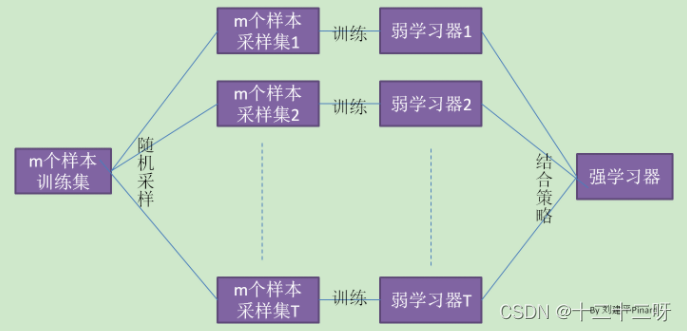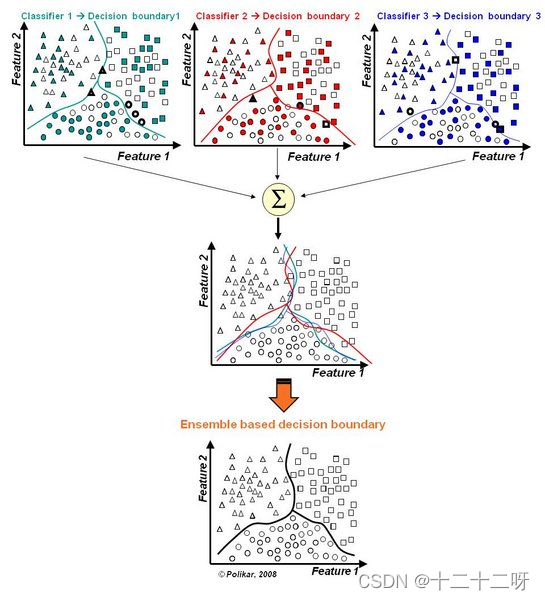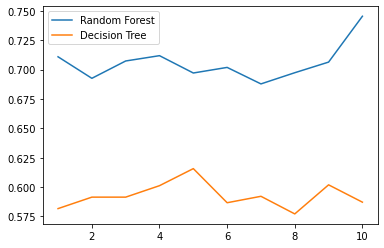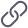• 随机森林分类python
2021-03-15 20:43:50

# 1 声明

本文的数据来自网络，部分代码也有所参照，这里做了注释和延伸，旨在技术交流，如有冒犯之处请联系博主及时处理。

# 2 决策树分类简介

相关概念见下：

决策树的最大问题是树在训练集生成的过于茂密，导致过拟合(即在训练集上表现卓越但在测试集上效果很差)。这时随机森林就应运而生了。在随机森林里会有很多决策树，而每颗决策树只接收自举样本且每个节点仅围绕部分特征寻找最好的分割。随机决策树的森林最后通过投票的方式选出最后的预测分类。

注：自举样本是指从一个较大的样本中 "自举 "出来的较小样本。Bootstrap是一种重新取样的方法，即从一个原始样本中反复抽取大量相同大小的小样本，并进行替换。

随机森林分类里的几个参数：

max_features：每个节点选择的特征的最大数

boostrap：指定是否以自举采样

n_estimators： 指定决策树的个数

n_jobs：并行数量，等于-1是最大化计算资源

# 3 随机森林分类代码与注释示例

# 加载随机森林分类包
from sklearn.ensemble import RandomForestClassifier
from sklearn import datasets
# 加载数据与模型创建
features = iris.data
target = iris.target
randomforest = RandomForestClassifier(random_state=0, n_jobs=-1)
# 训练模型
model = randomforest.fit(features, target)
# 预测分类
observation = [[ 5, 4, 3, 2]]
# Predict observation's class
print(model.predict(observation))

# 4 总结

更多相关内容
• 决策树对于数据分类Python实现，测试集和训练集分别给出，而且数据已经处理好的，
• 【项目实战】基于Python实现随机森林分类模型(RandomForestClassifier)项目 资料说明：包括数据集+源代码+Pdf文档说明。 资料内容包括： 1)项目背景； 2)获取数据； 3)数据预处理： (1)导入程序库并读取数据 ...
• python 随机森林分类 代码 #随机森林分类 import pandas as pd from sklearn.ensemble import RandomForestClassifier # 导入sklearn库的RandomForestClassifier函数 from sklearn.model_selection import train_...

python 随机森林分类 代码

#随机森林分类

import pandas as pd
from sklearn.ensemble import RandomForestClassifier  # 导入sklearn库的RandomForestClassifier函数
from sklearn.model_selection import train_test_split
from sklearn import metrics  # 分类结果评价函数

print(data)

'''
y    x1    x2    x3
0   1  0.697  0.460   0
1   1  0.774  0.376   0
2   1  0.634  0.264   0
3   1  0.608  0.318   0
4   1  0.556  0.215   0
5   1  0.403  0.237   1
6   1  0.481  0.149   1
7   1  0.437  0.211   1
8   0  0.666  0.091   1
9   0  0.243  0.267   2
10  0  0.245  0.057   2
11  0  0.343  0.099   2
12  0  0.639  0.161   0
13  0  0.657  0.198   0
14  0  0.360  0.370   1
15  0  0.593  0.042   2
16  0  0.719  0.103   1
'''

x = data[['x1', 'x2', 'x3']]  # 特征
y = data['y']  # 标签

# 划分数据集
x_train, x_test, y_train, y_test = train_test_split(x, y, random_state=0, train_size=0.8)
print('训练集和测试集 shape', x_train.shape, y_train.shape, x_test.shape, y_test.shape)

model = RandomForestClassifier()  # 实例化模型RandomForestClassifier
model.fit(x_train, y_train)  # 在训练集上训练模型
print(model)  # 输出模型RandomForestClassifier

# 在测试集上测试模型
expected = y_test  # 测试样本的期望输出
predicted = model.predict(x_test)  # 测试样本预测

# 输出结果
print(metrics.classification_report(expected, predicted))  # 输出结果，精确度、召回率、f-1分数
print(metrics.confusion_matrix(expected, predicted))  # 混淆矩阵

auc = metrics.roc_auc_score(y_test, predicted)
accuracy = metrics.accuracy_score(y_test, predicted)  # 求精度
print("Accuracy: %.2f%%" % (accuracy * 100.0))


展开全文• [ 2.] 2 2 在这里我有点困惑，就是在scikit-learn算法包中随机森林实际上就是一颗颗决策树组成的。但是之前我写的决策树博客中是可以将决策树给显示出来。但是随机森林却做了黑盒处理。我们不知道内部的决策树结构...

from sklearn.tree import DecisionTreeRegressor

from sklearn.ensemble import RandomForestRegressor

import numpy as np

#print iris#iris的4个属性是：萼片宽度　萼片长度　花瓣宽度　花瓣长度　标签是花的种类：setosa versicolour virginica

print iris['target'].shape

rf=RandomForestRegressor()#这里使用了默认的参数设置

rf.fit(iris.data[:150],iris.target[:150])#进行模型的训练

#

#随机挑选两个预测不相同的样本

instance=iris.data[[100,109]]

print instance

print 'instance 0 prediction；',rf.predict(instance)

print 'instance 1 prediction；',rf.predict(instance)

print iris.target,iris.target

返回的结果如下：

(150,)

[[ 6.3 3.3 6. 2.5]

[ 7.2 3.6 6.1 2.5]]

instance 0 prediction； [ 2.]

instance 1 prediction； [ 2.]

2 2

在这里我有点困惑，就是在scikit-learn算法包中随机森林实际上就是一颗颗决策树组成的。但是之前我写的决策树博客中是可以将决策树给显示出来。但是随机森林却做了黑盒处理。我们不知道内部的决策树结构，甚至连父节点的选择特征都不知道是谁。所以我给出下面的代码（这代码不是我的原创），可以显示的显示出所有的特征的贡献。所以对于贡献不大的，甚至是负贡献的我们可以考虑删除这一列的特征值，避免做无用的分类。

[python] view plain copy

from sklearn.cross_validation import cross_val_score, ShuffleSplit

X = iris["data"]

Y = iris["target"]

names = iris["feature_names"]

rf = RandomForestRegressor()

scores = []

for i in range(X.shape):

score = cross_val_score(rf, X[:, i:i+1], Y, scoring="r2",

cv=ShuffleSplit(len(X), 3, .3))

scores.append((round(np.mean(score), 3), names[i]))

print sorted(scores, reverse=True)

显示的结果如下：

[(0.934, 'petal width (cm)'), (0.929, 'petal length (cm)'), (0.597, 'sepal length (cm)'), (0.276, 'sepal width (cm)')]

这里我们会发现petal width、petal length这两个特征将起到绝对的贡献，之后是sepal length，影响最小的是sepal width。这段代码将会提示我们各个特征的贡献，可以让我们知道部分内部的结构。

展开全文• 随机森林是bagging的一个特化进阶版 特化是指：随机森林的弱学习器都是决策树 进阶是指：在bagging样本随机采样的基础上，又加上了特征的随机选择 Bagging是一种有放回的重复抽样方法，各学习器之间没有依赖关系...

## 知识点：

• 集成模型（Ensemble）的思想：为了解决单个模型所固有的缺陷，从而整合更多的模型，取长补短，避免局限性。
• 自助法（Bootstrap）：即从样本自身中再生成很多可用的同等规模的新样本，从自己中产生和自己类似的，所以叫做自助，即不借助其他样本数据。（有放回的抽样问题）
• bagging的名称来源于 （ Bootstrap AGGregatING ），意思是自助抽样集成，这种方法将训练集分成m个新的训练集，然后在每个新训练集上构建一个模型，各自不相干，最后预测时我们将这个m个模型的结果进行整合，得到最终结果
• 对于分类：通常使用简单投票法，得到最多票数的类别或者类别之一为最终的模型输出
• 对于回归：通常使用简单平均法，对T个弱学习器得到的回归结果进行算术平均得到最终的模型输出。（分类投票，回归均值）
• 随机森林是bagging的一个特化进阶版
• 特化是指：随机森林的弱学习器都是决策树
• 进阶是指：在bagging样本随机采样的基础上，又加上了特征的随机选择
• Bagging是一种有放回的重复抽样方法，各学习器之间没有依赖关系，可以并行生成
• Boosting各个学习器之间是串联的关系，每一轮的训练集不变，改变的是样本的权重
• Bagging+决策树=随机森林
• 随机森林的构造过程：行采样和列采样
• 行采样：假设有N个样本，有放回的随机选择N个样本（自助法）
• 列采样：每个样本有M个属性，随机选择m个属性，m<<M，然后从这m个属性中采用某种策略（信息增益）来选择1个属性作为该节点的分裂属性
• bagging每轮随机采样中，训练集大约有36.8%的数据没被采样，被称为“袋外数据”
• m是决策树的重要参数，m越小，方差越小，拟合程度越差，偏倚越大，一般选择交叉验证法寻找最佳m
• 整个决策树形成过程中没有进行剪枝，因为特征和样本的随机性选择导致不容易陷入过拟合
• 随机森林具有很好的抗噪声能力
• 能处理很高维（feature很多）的数据，并且不用做特征选择
• 可以度量样本之间的相似性
• 训练过程中能检测特征重要性，是常见的特征筛选方法。当某一特征在所有树种离树根的平均距离越近，这一特征就越重要
• 需要调整的参数有：决策树个数，递归次数（决策树深度），特征选择的个数
• 决策树个数：越多越稳定
• 决策树深度：深度越小，计算量越小，速度越快，常用的取值为10-100
• 特征选择的个数：减少特征选择个数，不仅会提升算法个数，也可能降低测试误差，通常使用的值为## sklearn参数：

Random Forest算法参数解释及调优_nobody~的博客-CSDN博客_随机森林参数调优

### A.使模型预测更好的特征：主要有3类特征可以被调整，以改善该模型的预测能力

1.max_features:允许单个决策树使用特征的最大数量，Python为最大特征数提供了多个可选项:

• Auto/None ：简单地选取所有特征，每颗树都可以利用他们
• sqrt ：此选项是每颗子树可以利用总特征数的平方根个。 例如，如果变量（特征）的总数是100，所以每颗子树只能取其中的10个
• 0.X：此选项允许每个随机森林的子树可以利用变量（特征）数的X0％
• 增加max_features一般能提高模型的性能，因为在每个节点上，我们有更多的选择可以考虑。 然而，这未必完全是对的，因为它降低了单个树的多样性，而这正是随机森林独特的优点。 但是，可以肯定，你通过增加max_features会降低算法的速度。 因此，你需要适当的平衡和选择最佳max_features

2.n_estimators:在利用最大投票数或平均值来预测之前，你想要建立子树的数量

• 较多的子树可以让模型有更好的性能，但同时让你的代码变慢。 你应该选择尽可能高的值，只要你的处理器能够承受的住，因为这使你的预测更好更稳定。

3.min_sample_leaf:最小样本叶片大小。

• 叶是决策树的末端节点。 较小的叶子使模型更容易捕捉训练数据中的噪声。 一般来说，我更偏向于将最小叶子节点数目设置为大于50。在你自己的情况中，你应该尽量尝试多种叶子大小种类，以找到最优的那个

### B.使得模型训练更容易的特征

1.n_jobs:这个参数告诉引擎有多少处理器是它可以使用。

• “-1”意味着没有限制，而“1”值意味着它只能使用一个处理器

2.random_state:此参数让结果容易复现。

## 采用工具包实现随机森林和单个决策树：

数据集来源：

https://archive.ics.uci.edu/ml/machine-learning-databases/undocumented/connectionist-bench/sonar/

import csv
from sklearn.model_selection import train_test_split
from sklearn.tree import DecisionTreeClassifier # 决策树
from sklearn.ensemble import RandomForestClassifier # 随机森林

'''加载数据，一行行的存入列表'''
dataSet = []
with open(filename, 'r') as file:
dataSet.append(line)
return dataSet

'''处理数据，除了标签列，其他列都转换为float类型'''
def column_to_float(dataSet):
featLen = len(dataSet) - 1
for data in dataSet:
for column in range(featLen):
data[column] = float(data[column].strip())
column_to_float(dataSet)

'''划分数据集7：3'''
X = []
Y = []
for i in dataSet:
x= i[:-1]
y = i[-1]
Y.append(y)
X.append(x)
X_train, X_test, y_train, y_test = train_test_split(X,Y, test_size=0.3)

'''计算准确率'''
def accuracy(predict_values, actual):
correct = 0
for i in range(len(actual)):
if actual[i] == predict_values[i]:
correct += 1
return correct / float(len(actual))

'''工具包预测结果'''
rf=RandomForestClassifier(random_state=0)# 随机森林
clf = DecisionTreeClassifier(random_state=0)# 单个决策树
rf = rf.fit(X_train,y_train)
clf = clf.fit(X_train,y_train)
score_rf =rf.score(X_test,y_test)
score_clf =clf.score(X_test,y_test)
print("随机森林：",score_rf)
print("决策树：",score_clf)

实验结果：

随机森林： 0.8412698412698413
决策树： 0.6349206349206349

画出随机森林和决策树在十组交叉验证下的效果对比

画出随机森林和决策树在十组交叉验证下的效果对比
rfc_l = []
clf_l = []
for i in range(10):
rfc = RandomForestClassifier(n_estimators=25)
rfc_s = cross_val_score(rfc,X,Y,cv=10).mean()
rfc_l.append(rfc_s)
clf = DecisionTreeClassifier()
clf_s = cross_val_score(clf,X,Y,cv=10).mean()
clf_l.append(clf_s)

plt.plot(range(1,11),rfc_l,label = "Random Forest")
plt.plot(range(1,11),clf_l,label = "Decision Tree")
plt.legend()
plt.show()## 详细代码实现随机森林（来自网友）

import csv
from random import seed
from random import randrange
from math import sqrt

'''决定输出标签'''
def decide_label(data):
output = [row[-1] for row in data]
# 简单投票法
return max(set(output), key=output.count)

'''子分割，不断地构建叶节点的过程'''
def sub_spilt(root, n_features, max_depth, min_size, depth):
left = root['left']
right = root['right']
del (root['left'])
del (root['right'])
if not left or not right:
root['left'] = root['right'] = decide_label(left + right)
return
if depth > max_depth:
root['left'] = decide_label(left)
root['right'] = decide_label(right)
return
if len(left) < min_size:
root['left'] = decide_label(left)
else:
root['left'] = get_best_spilt(left, n_features)
sub_spilt(root['left'], n_features, max_depth, min_size, depth + 1)
if len(right) < min_size:
root['right'] = decide_label(right)
else:
root['right'] = get_best_spilt(right, n_features)
sub_spilt(root['right'], n_features, max_depth, min_size, depth + 1)

'''计算分割代价'''
def spilt_loss(left, right, class_values):
loss = 0.0
for class_value in class_values:
left_size = len(left)
if left_size != 0:  # 防止除数为零
# list.count(obj)返回元素在列表中出现的次数。
prop = [row[-1] for row in left].count(class_value) / float(left_size)
loss += (prop * (1.0 - prop))# GINI指数
right_size = len(right)
if right_size != 0:
prop = [row[-1] for row in right].count(class_value) / float(right_size)
loss += (prop * (1.0 - prop))
return loss

'''分割数据集,左右分支'''
def data_spilt(dataSet, index, value):
left = []
right = []
for row in dataSet:
if row[index] < value:
left.append(row)
else:
right.append(row)
return left, right

'''选取任意的n个特征，在这n个特征中，选取分割时的最优特征'''
def get_best_spilt(dataSet, n_features):
features = []
class_values = list(set(row[-1] for row in dataSet))
b_index, b_value, b_loss, b_left, b_right = 999, 999, 999, None, None
# 列采样
while len(features) < n_features:
index = randrange(len(dataSet)-1)
if index not in features:
features.append(index)
for index in features:#找到列的最适合做节点的索引，（损失最小）
for row in dataSet:
left, right = data_spilt(dataSet, index, row[index])#以它为节点的，左右分支
loss = spilt_loss(left, right, class_values)# 计算分割代价
if loss < b_loss:#寻找最小分割代价
b_index, b_value, b_loss, b_left, b_right = index, row[index], loss, left, right
return {'index': b_index, 'value': b_value, 'left': b_left, 'right': b_right}

'''构造决策树'''
def build_tree(dataSet, n_features, max_depth, min_size):
root = get_best_spilt(dataSet, n_features)
sub_spilt(root, n_features, max_depth, min_size, 1)
return root

'''行采样，随机抽样选择N个样本'''
def get_subsample(dataSet, ratio):
subdataSet = []
lenSubdata = round(len(dataSet) * ratio)#四舍五入保留整数
while len(subdataSet) < lenSubdata:
index = randrange(len(dataSet))# 行采样，随机选择
subdataSet.append(dataSet[index])
return subdataSet

'''预测测试集结果'''
def predict(tree, row):
predictions = []
if row[tree['index']] < tree['value']:
if isinstance(tree['left'], dict):
return predict(tree['left'], row)
else:
return tree['left']
else:
if isinstance(tree['right'], dict):
return predict(tree['right'], row)
else:
return tree['right']
def bagging_predict(trees, row):
predictions = [predict(tree, row) for tree in trees]
return max(set(predictions), key=predictions.count)

'''创建随机森林'''
def random_forest(train, test, ratio, n_feature, max_depth, min_size, n_trees):
trees = []
for i in range(n_trees):
train = get_subsample(train, ratio)#从切割的训练集中选取子集
tree = build_tree(train, n_features, max_depth, min_size)#构建 一个决策树
# 随机森林
trees.append(tree)

predict_values = [bagging_predict(trees, row) for row in test]
return predict_values

'''将数据集随机分成n_folds块，方便交叉验证，其中一块是测试集，其他四块是训练集'''
def spiltDataSet(dataSet, n_folds):
fold_size = int(len(dataSet) / n_folds)
dataSet_copy = list(dataSet)
dataSet_spilt = []
for i in range(n_folds):
fold = []
while len(fold) < fold_size:  # 这里不能用if，if只是在第一次判断时起作用，while执行循环，直到条件不成立
index = randrange(len(dataSet_copy))
fold.append(dataSet_copy.pop(index))  # pop() 函数用于移除列表中的一个元素（默认最后一个元素），并且返回该元素的值。
dataSet_spilt.append(fold)
return dataSet_spilt

'''加载数据，一行行的存入列表'''
dataSet = []
with open(filename, 'r') as file:
dataSet.append(line)
return dataSet

'''除了标签列，其他列都转换为float类型'''
def column_to_float(dataSet):
featLen = len(dataSet) - 1
for data in dataSet:
for column in range(featLen):
data[column] = float(data[column].strip())

'''计算准确率'''
def accuracy(predict_values, actual):
correct = 0
for i in range(len(actual)):
if actual[i] == predict_values[i]:
correct += 1
return correct / float(len(actual))

n_folds = 5# 划分数据集数，用于后续交叉验证
n_features = 7# 选取特征数
n_trees = 20# 决策树数量
max_depth = 15
min_size = 1
ratio = 1.0
scores = []
folds = spiltDataSet(dataSet, n_folds)
# 该部分选取4个数据集作为训练集，1个数据集作为测试集，实现交叉验证
for fold in folds:
train_set = folds[:]
train_set.remove(fold)
train_set = sum(train_set, [])
test_set = []
for row in fold:
row_copy = list(row)
row_copy[-1] = None
test_set.append(row_copy)
actual = [row[-1] for row in fold]
predict_values = random_forest(train_set, test_set, ratio, n_features, max_depth, min_size, n_trees)
accur = accuracy(predict_values, actual)
scores.append(accur)
print ('Trees is %d' % n_trees)
print ('scores:%s' % scores)
print ('mean score:%s' % (sum(scores) / float(len(scores))))

输出：

Trees is 20
scores:[0.7804878048780488, 0.6341463414634146, 0.4878048780487805, 0.7560975609756098, 0.8048780487804879]
mean score:0.6926829268292682

学习笔记参考以下博客

机器学习：04. 随机森林之RandomForestClassifier - 简书https://www.jianshu.com/p/c1afbe4b9364

展开全文机器学习 数据挖掘 算法
• 1.前言：本实验采用的是GSR数据，机器学习方法为随机森林 2.GSR数据如下图所示： 3.随机森林代码： import numpy as np import pandas as pd from sklearn.model_selection import train_test_split from ...
• 使用Python进行随机森林图像分类 使用Python随机森林图像分类 请遵循以下文件夹结构。 图像分类（文件夹） 数据集（文件夹） 火车（文件夹） 图像Cat1文件夹 train_img.jpg train_img.jpg train_img.jpg .....
• 数学建模_随机森林分类模型详解Python代码 随机森林需要调整的参数有： （1） 决策树的个数 （2） 特征属性的个数 （3） 递归次数（即决策树的深度）''' from numpy import inf from numpy import zeros import ...决策树 机器学习 深度学习 人工智能
• 随机森林 分类模型iris_rForest.py from sklearn import datasets from sklearn.metrics import confusion_matrix,accuracy_score from sklearn.model_selection import train_test_split from sklearn import ...机器学习
• 机器学习算法（8）python实现随机森林分类） 可以把随机森林看成是决策树的集 合。随机森林背后的逻辑是对分别受较大方差影响的多个决策树取平均值， 以建立一个具有更好的泛化性能和不易过拟合的强大模型。 ...机器学习 决策树
• 原标题：随机森林的原理及Python代码实现最近在做kaggle的时候，发现随机森林这个算法在分类问题上效果十分的好，大多数情况下效果远要比svm，log回归，knn等算法效果好。因此想琢磨琢磨这个算法的原理。要学随机...
• 随机森林也被称为随机决策森林，是一种集合学习方法，既可以用于分类，也可以用于回归。 随机森林把不同的几棵决策树打包到一块，每棵树的参数都不相同，然后把每棵树预测的结果取平均值，这样既保留决策树们的工作...算法
• ## Python 中的随机森林

千次阅读 2022-01-04 22:27:15
什么是随机森林 众所周知，树模型是高方差、低偏差的模型。因此，它们容易过度拟合训练数据。如果我们不修剪树模型或引入早期停止标准（例如每个叶节点的最小实例数），我们可以概括一下树模型的作用，这很吸引人...机器学习
• 1.4 什么是随机森林？ 二、Random Forest 的构造过程 2.1 算法实现 2.2数据的随机选取 2.3待选特征的随机选取 2.4 相关概念解释 三、 Random Forest 优缺点 3.1 优点 3.2 缺点 四、Extra-Trees（极端随机树...算法
• python编写的随机森林分类器。 用法 from sklearn . datasets import load_digits from sklearn import cross_validation import numpy as np from randomforest import RandomForestClassifier digits = load_...
• 本项目使用了决策树和随机森林2种机器学习方法进行实验，完整代码在最下方，想要先看源码的同学可以移步本文最下方进行下载。 博主也参考过文本分类相关模型的文章，但大多是理论大于方法。很多同学肯定对原理不需要...
• 本文从单棵决策树讲起，然后逐步解释了随机森林的工作原理，并使用sklearn中的随机森林对某个真实数据集进行预测。如今由于像Scikit-Learn这样的库的出现，我们可以很容易地在Python中实现数百种机器学习算法。它们...
• 文字部分： ... 数学的东西直接看代码。 ########################################################################## ...下面是给了数据集之后，训练随机森林的过程： 首先，咱们先来看一棵树的成长！确定好这棵...机器学习算法
• 随机森林（Random forest, RF）是 Breiman 教授在 2001 年提出的集成学习方法，是一种统计学习理论。它是基于装袋法Bagging集成理论实现的，利用自助法 bootstrap抽样技术从原始数据集中有放回地抽取多个不同的训练...
• 主要介绍了Python实现的随机森林算法,结合实例形式详细分析了随机森林算法的概念、原理、实现技巧与相关注意事项,需要的朋友可以参考下
• 文章目录1. 数据准备2. 相关代码3. 结果展示 1. 数据准备 遥感影像 n个shp文件（n为类别数，其中包括背景类） 截图如下 2. 相关代码 # -*- coding: utf-8 -*- import os, sys, time ...from skimage impo
• ## python实现随机森林

万次阅读 多人点赞 2022-01-27 12:21:56机器学习
• 利用Python的Scikit-Learn库对遥感影像进行随机森林(RandomForest)分类 ...因此，本篇文章将为读者介绍利用Python及其扩展包Scikit-Learn对遥感影像进行随机森林分类的完整过程，包括：ShapeFile格式样本数据的读取、
• 说明：这是一个机器学习实战项目（附带数据+... 预测结果数据如下： 本次机器学习项目实战所需的资料，项目资源如下： 基于Python实现随机森林分类模型(RandomForestClassifier)项目实战-Python文档类资源-CSDN下载机器学习 项目实战
• Python-回归-树-森林回归树和随机森林Python 实现。 参见 Breiman 等人的“分类和回归树”。 (1984)。 Regression_tree_cart.py 模块包含在给定一些训练数据的情况下增长和使用回归树的函数。 Football_parserf....
• 本文将介绍如何使用Python构建和使用随机森林。我们不只是简单地展示代码，而会尽力解释模型的工作方式。我们将从一个解决简单问题的单个决策树开始，然后逐渐深入，最终完成一个针对某个真实世界数据科学问
• 本文实例讲述了Python决策树和随机森林算法。分享给大家供大家参考，具体如下： 决策树和随机森林都是常用的分类算法，它们的判断逻辑和人的思维方式非常类似，人们常常在遇到多个条件组合问题的时候，也通常可以画...
• Python:实现random forest classifier随机森林分类器算法(附完整源码)算法...